### Computing Average Monthly Flow Values using Microsoft Access

In Water Resources Engineering, the analysis of period of record data is often performed to provide a summary of the conditions at a particular site.  In this analysis, variables such as the overall average, average by month, minimum value, maximum value, etc. can be determined.  In this post, the average by month will be computed.  The data used is USGS flow data for the White River at Newport.  This gage is located in the state of Arkansas.  The period of record extends back to 1927.  For this analysis, the data set has 33,928 values.  Some of the dates have missing data.  This data set was previously analyzed using Microsoft Excel.  In that analysis, the average value for the month of July was computed to be 16,758.45 cfs when accounting for the missing data (meaning that missing data is excluded from the computation of the average rather than having a value of zero assigned to them).  That analysis is shown in the following post:

Step 1:

The first step involved importing the flow data into the Access database.  The image below shows a small portion of the table.  The table is titled, "Newport flow data",

Step 2:

From the menu, select "Create --> Query Design".  We then drag the Newport flow data into the gray space.  This allows us to select items from the table to be used in the query.

Step 3:

In this step, date and flow are selected as the fields from the table titled, "Newport flow data".

Step 4:

From the menu, select the sum totals button (as shown below) to be able to access the ability to compute averages.  Note that you must be in Design View to access this option.

Step 5:

Since the flow data is the variable we are using to compute the average, "Avg" is selected in the "Total" row and the "flow" field.

Step 6:

If we were to use this query as shown above, we would get the average of all of the flow values for the period of record.  However, we are looking for the average monthly flow values instead.  In order to get this, we need to make a slight modification to the date field to let Access know that we are wanting the average by month.  In the date field we change "date" to "format(date,'mm') ".  The 'mm' is used to let Access know that we are using a two value numeric format for the month in our date.  The edited field is shown below.

Step 7:

Now that we have the query developed, we move from Design View to Datasheet View to view the results of the query (this is done from the View menu).  In the results shown below, the July average of 16,758.45 matches the value obtained when the data was analyzed using Excel.  This value confirms that Access is not incorporating missing data into the computation of the average value.

### Computing Average Monthly Flow Values using Excel and Visual Basic

In the field of water resources engineering, it is often necessary to perform data analysis on time-series data.  For example, a user of this data may want to summarize average monthly flow data to examine the month or season when flows are the highest along with determining the difference between the average monthly high flow and the average monthly low flow.  This can be done using a variety of methods.  Below, methods for doing this directly in Microsoft Excel and for using Visual Basic in Microsoft Excel are shown.

For this example, daily average USGS flow data for the White River at Newport, Arkansas is used.  The data ranged from 1927 to 2020.  A small portion of the data is shown below.  The date is given in column A while the flow value is given in column B.  This data set has close to 34,000 values.

To perform this analysis, all of the July values are extracted and put into column C.  To do this, a simple “IF” statement in column C is used for all of the rows of data.  The “IF” statement is as follows:

=IF(MONTH(A2)=7,B2,"")

This “IF” statement indicates that if the month in column A has a numeric value of 7 for July, then column C is populated with the flow value found in column B for that row.  If it is not the month of July, then that row in column C is to be empty.  After doing that, the minimum, maximum, and average values in column C are computed.

 avg 15677.3 min 0 max 92600

In reviewing the data, the minimum value is found to be zero, which does not seem to be a reasonable value since a daily average flow value of zero at this location in the White River is unlikely.  This points to the importance of reviewing data prior to an analysis.  In examining the data, it becomes obvious that the method that Excel is using for missing data based on the “IF” statement is incorrect.  Observation of the values in July 1932 confirms this.  This data is missing for this month; however, Excel is outputting zero values based on the analysis of the “IF” statement.

To solve this issue, an adjustment to the “IF” statement is required.  An additional check is added to the “IF” to check whether the flow value is blank.  This is shown below.  Now, the two conditions of the month being July and the flow value not being empty must be satisfied to populate the column.  Doing this removes the zero values which were adding nothing to the running sum of the flows, but they were increasing the number of values.  This had the effect of reducing the true average.

=IF(AND(MONTH(A2)=7,NOT(B2="")),B2,"")

Using this updated “IF” statement and re-computing the average, minimum, and maximum values gives the following:

 avg 16758.5 min 4180 max 92600

As expected, the average value increases since the zero values are no longer counted and the minimum value is no longer zero.

The computation of this in Excel is relatively straightforward, but Visual Basic within Excel can also be used.  The first code that is shown reproduces the example where missing values still add to the count.  In this code, the variable “Monthnum” is set as an integer.  A running sum and a count of July values is used to compute the average.  The code begins in cell A1, which is a header cell.  The code obtains the date value and the flow value for each time step by using the offset command to move through the values.  The “Do” loop is used to continue the operation until it runs out of date values.  It should be noted that if there are blank values in the date column, this would need to be cleaned up for the code to work as intended.

Once this code is simulated, the average value for July is output to cell “F11”.  The computed value for this code is 15677.26, which matches the average found using the first “IF” statement shown earlier.  The answers are the same since this code suffers from the same issues as the earlier “IF” statement.

The solution is to once again alter the IF statement to account for empty values.  The rest of the code remains the same.  The updated code is shown below.

Adding the “IsEmpty” check solves the issue and gives the correct average of 16758.45.

This analysis demonstrates straightforward methods for determining monthly average values using Excel and using Excel with Visual Basic.  It also shows the importance of examining data prior to an analysis along with the importance of reviewing results provided by the chosen method.

### Comparison of Time-Series Data Analysis

In this post, I examine a few different methodologies for determine the largest flow events from a time series of flow data at Newport, Arkansas on the White River.  The analysis uses daily average flow data from the USGS.  For this analysis, I want not only the highest flow values, but I also want the date that is associated with those values.

This flow data can be found in the following location:

The image below indicates that the flow data is available from 1927 to the present.  I select to get the data in a Tab-separated file.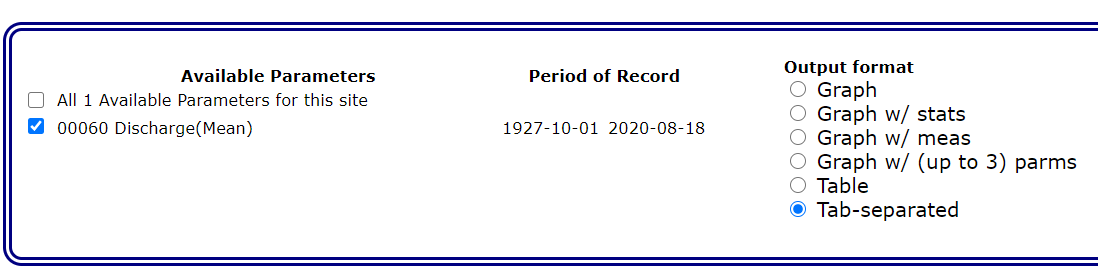Method #1:  Using Excel to Sort the Data

Probably the easiest and most direct method for performing this analysis is to import the data into Excel and then sort by the flow values.  You need to sort the entire table to ensure that the proper date is still linked to the corresponding flow.  After importing the data and sorting by flow value from largest to smallest, the top 15 values are shown below.  The largest value of 340,000 cfs occurred on April 18, 1945.  You can see that some of the other top values are also associated with this April 1945 event.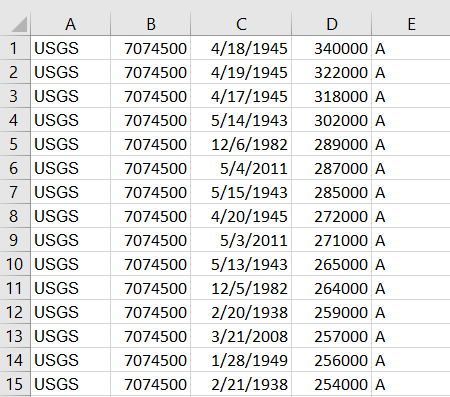Method #2:  Using USGS to Obtain the Annual Peaks

If you are only interested in the annual peaks, USGS will typically have those values tabulated.  It should be noted, however, that peak streamflow values from USGS can be instantaneous values, which are likely to vary from the daily values.  It should also be noted that instantaneous data set may contain a different period of record than the daily data set.  In the data set below, you can see that the peak streamflow data begins in the year 1886 for this site.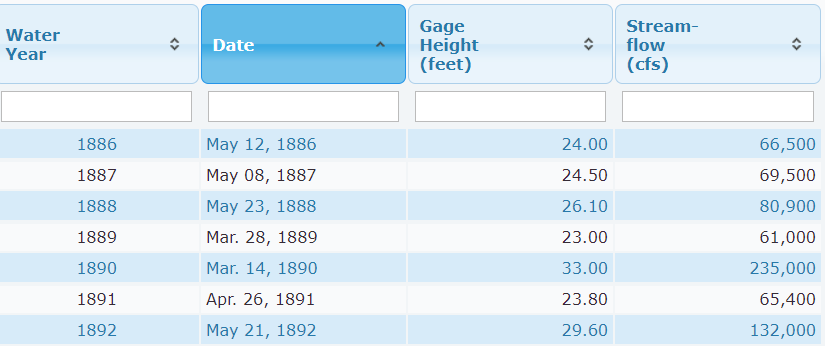On the USGS site, we can sort the streamflow values from highest to lowest by clicking on that column heading.  The first click appears to order them from lowest to highest.  Clicking on it again orders them from highest to lowest.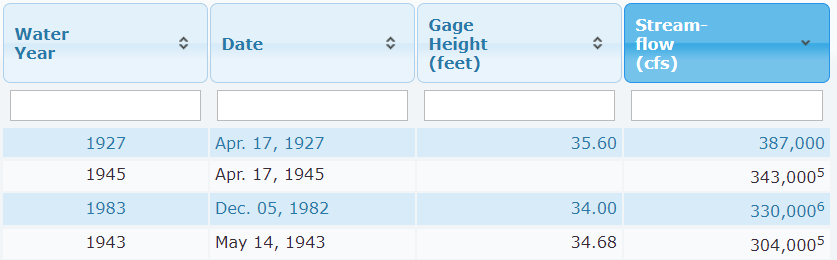In the image above, two items are apparent in the data.  The first is that peak recorded flow is 387,000 cfs on April 17, 1927.  This value did not appear in the daily values since the daily values began in October 1927.  The next is that the peak flow in the April 1945 event is recorded as 343,000 cfs as opposed to the 340,000 cfs that was found using the daily data.  It is important to provide this type of context whenever you are summarizing time series data.

Method #3:  Using HEC-DSSVue

HEC-DSSVue is a Corps of Engineers database that is well suited for handling time series data.  To use HEC-DSSVue for this analysis, I first import the data.  I was unable to get the direct import from USGS to work so I copied the data from an Excel spreadsheet using the manual data entry in HEC-DSSVue.  I now have a full time series of the daily data.  If I want to get the peak streamflow for each year, I can use the Math Functions within HEC-DSSVue.

To access this, I select Tools > Math Functions.  I then select Time Functions.  I select the Maximum for Period.  I select Water Year as the New Period Interval.  Since I want to preserve the date of the maximum value, I also select Save as Irregular Interval with the Block Size being IR-YEAR (meaning irregular values for each year as opposed to using the end of the water year as the date for each year).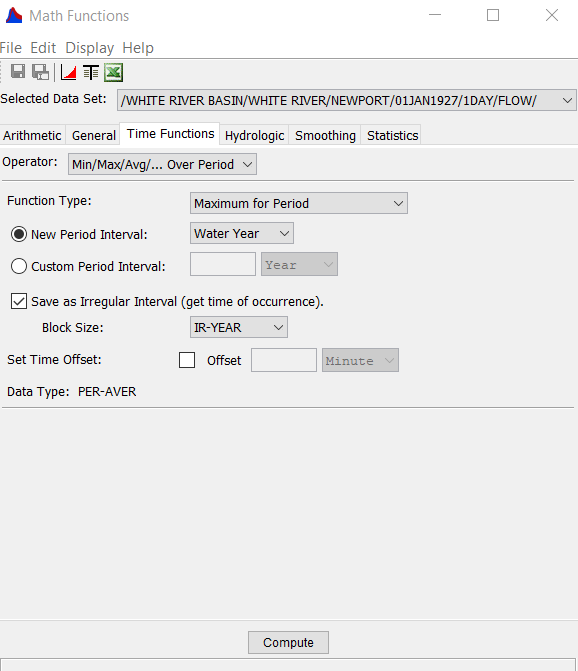HEC-DSSVue creates an additional path that can be sorted by clicking on the header of the flow column.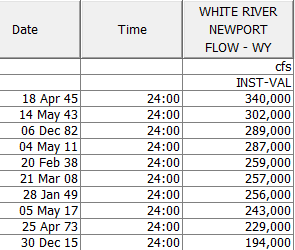Method #2 and Method #3 are similar in that they give peak annual values.  Method #2 gave peak instantaneous values while Method #3 gave peak daily values.  Method #1 gave peak daily values that were not limited to one value per year.

Method #4:  Python Script to Extract Largest Daily Flow Values

Since the USGS website and HEC-DSSVue are set up for this type of data, I would prefer to use those sources or to use Excel for data sorting if I needed multiple values within a given year.  However, it is possible to read text files using Python and to print a desired result.  In this example, I use Python to print out values that exceed 100,000 cfs.  It should be noted that I shortened the data set significantly for testing.  The data set is shown below.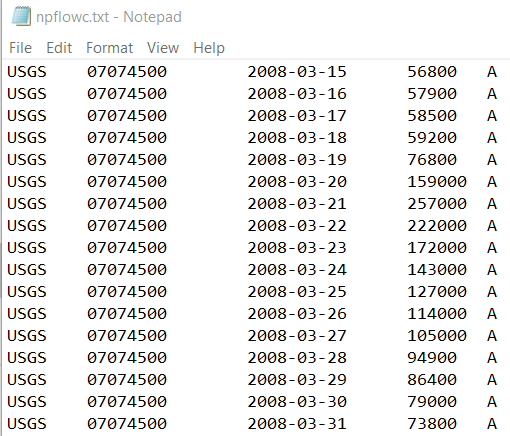In the Python code below, I import the module named csv.  I use that to read each line in the text file as a list by indicating that the items are tab delimited.  I then select the flow column, which has an index of 3 since the first item in a Python list has the index of zero, and test if the value is above 100,000 cfs.  If it is, then print the date and flow value are printed.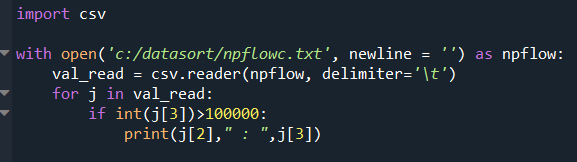The result of this code is shown below.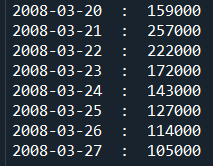## Table Rock Dam